## Mechanical Properties of Solids Questions and Answers Part-13

1. The adiabatic elasticity of a gas is equal to
a) $\gamma\times density$
b) $\gamma\times volume$
c) $\gamma\times pressure$
d) $\gamma\times$  specific heat

Explanation: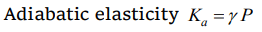2. The specific heat at constant pressure and at constant volume for an ideal gas are $C_{p}$ and $C_{v}$ and its adiabatic and isothermal elasticities are $E_{\phi}$ and $E_{\theta}$ respectively. The ratio of $E_{\phi}$ to $E_{\theta}$
a) $C_{v}\diagup C_{p}$
b) $C_{p}\diagup C_{v}$
c) $C_{p} C_{v}$
d) $1\diagup C_{p} C_{v}$

Explanation: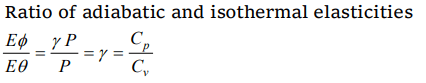3. The only elastic modulus that applies to fluids is
a) Young's modulus
b) Shear modulus
c) Modulus of rigidity
d) Bulk modulus

Explanation: Bulk modulus

4. The ratio of the adiabatic to isothermal elasticities of a triatomic gas is
a) 3/4
b) 4/3
c) 1
d) 5/3

Explanation: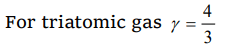5. If the volume of the given mass of a gas is increased four times, the temperature is raised from 27°C to 127°C. The elasticity will become
a) 4 times
b) 1/4 times
c) 3 times
d) 1/3 times

Explanation:6. If a rubber ball is taken at the depth of 200 m in a pool, its volume decreases by 0.1%. If the density of the water is $1\times 10^{3}kg\diagup m^{3}$  and $g= 10 m\diagup s^{2}$  , then the volume elasticity in $N\diagup m^{2}$ will be
a) $10^{8}$
b) $2\times10^{8}$
c) $10^{9}$
d) $2\times10^{9}$

Explanation:7.The compressibility of a material is
a) Product of volume and its pressure
b) The change in pressure per unit change in volume strain
c) The fractional change in volume per unit change in pressure
d) None of the above

Explanation: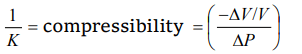8. When a pressure of 100 atmosphere is applied on a spherical ball, then its volume reduces to 0.01%. The bulk modulus of the material of the rubber in $dyne\diagup cm^{2}$  is
a) $10\times10^{12}$
b) $100\times10^{12}$
c) $1\times10^{12}$
d) $20\times10^{12}$

Explanation: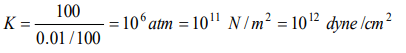9. In the three states of matter, the elastic coefficient can be
a) Young's modulus
b) Coefficient of volume elasticity
c) Modulus of rigidity
d) Poisson's ratio